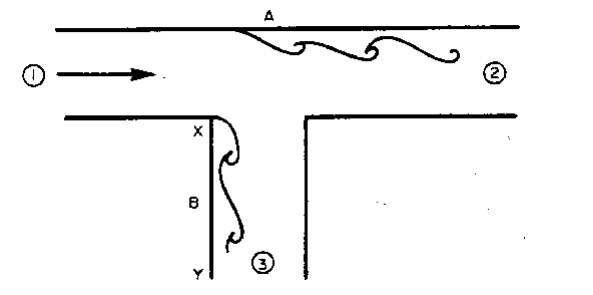Home | | Mechanics of Fluids | Mechanics Of Fluidsr: Boundary layer separation

# Mechanics Of Fluidsr: Boundary layer separation(i) Convergent flows: Negative pressure gradients (ii) Divergent flows: Positive pressure gradients (iii) A divergent duct or diffuser (iv) Tee-Junctions (v) Y-Junctions

Boundary layer separation

(i) Convergent flows: Negative pressure gradients

If flow over a boundary occurs when there is a pressure decrease in the direction of flow, the fluid will accelerate and the boundary layer will become thinner.

This is the case for convergent flows.The accelerating fluid maintains the fluid close to the wall in motion. Hence the flow remains stable and turbulence reduces. Boundary layer separation does not occur.

(ii) Divergent flows: Positive pressure gradients

When the pressure increases in the direction of flow the situation is very different. Fluid outside theboundary layer has enough momentum to overcome this pressure which is trying to push it backwards. The fluid within the boundary layer has so little momentum that it will very quickly be brought to rest,and possibly reversed in direction. If this reversal occurs it lifts the boundary layer away from the surfaceas shown below.This phenomenon is known as  boundary layer separation.

At the edge of the separated boundary layer, where the velocities change direction, a line of vortices occur (known as a vortex sheet). This happens because fluid to either side is moving in the opposite direction.This boundary layer separation and increase in the turbulence because of the vortices results in very large energy losses in the flow.

These separating / divergent flows are inherently unstable and far more energy is lost than in parallel or convergent flow.

(iii) A divergent duct or diffuser

The increasing area of flow causes a velocity drop (according to continuity) and hence a pressure rise(according to the Bernoulli equation).Increasing the angle of the diffuser increases the probability of boundary layer separation. In a Venturi meter it has been found that an angle of about 6 o provides the optimum balance between length of meter and danger of boundary layer separation which would cause unacceptable pressure energy losses.

(iv) Tee-JunctionsAssuming equal sized pipes, as fluid is removed, the velocities at 2 and 3 are smaller than at 1, the entrance to the tee. Thus the pressure at 2 and 3 are higher than at 1. These two adverse pressure gradients can cause the two separations shown in the diagram above.

(v) Y-Junctions

Tee junctions are special cases of the Y-junction with similar separation zones occurring. See the diagram below.Downstream, away from the junction, the boundary layer reattaches and normal flow occurs i.e. the effect of the boundary layer separation is only local. Nevertheless fluid downstream of the junction will have lost energy.

Study Material, Lecturing Notes, Assignment, Reference, Wiki description explanation, brief detail
Civil : Mechanics Of Fluids : Boundary Layer : Mechanics Of Fluidsr: Boundary layer separation |